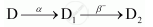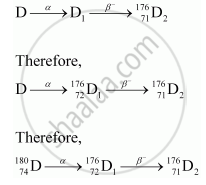Department of Pre-University Education, KarnatakaPUC Karnataka Science Class 12

# The Sequence of Stepwise Decay of a Radioactive Nucleus is If the Atomic Number and Mass Number of D2 Are 71 and 176 Respectively, What Are Their Corresponding Values of D? - Physics

#### Question

The sequence of stepwise decay of a radioactive nucleus isIf the atomic number and mass number of D2 are 71 and 176 respectively, what are their corresponding values of D?

#### SolutionSo, the corresponding values of atomic number and mass number for D are 74 and 180.

Is there an error in this question or solution?